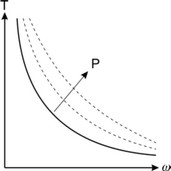﻿ 20-sim webhelp > Toolboxes > Mechatronics Toolbox > Servo Motor Editor > Theory > Torque Speed Plot > Limits

# Limits

Navigation:  Toolboxes > Mechatronics Toolbox > Servo Motor Editor > Theory > Torque Speed Plot >

# LimitsTo find out if a motor is sufficient, we have to plot the motor limits in the torque speed plot. In the previous chapter we have described the following limits:

 Limit Brush DC Brushless DC AC Synchronous torque / current V V V speed V V V power V X X voltage V X X

## Maximum Voltage

For higher voltages, a higher stall torque and no load speed is found but the steepness does not change.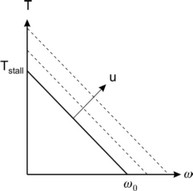## Maximum Torque / Maximum Current

In many data sheets a maximum torque is given. This is directly connected with a maximum current limit through the equation: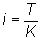So both the maximum current and the maximum torque appear as horizontal lines in the torque speed plot.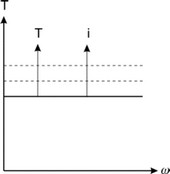## Maximum Velocity

The maximum velocity can be directly indicated in the torque speed plot as a vertical line.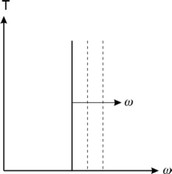## Maximum Power

The line of maximum power can be indicated as a parabola in the torque speed plot.